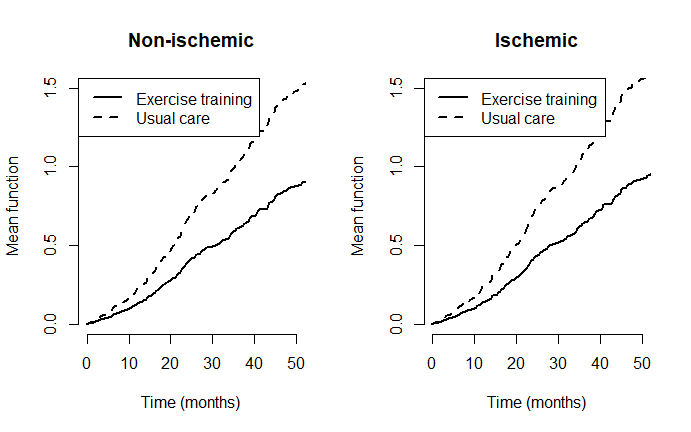# Proportional means regression analysis of weighted composite endpoint of recurrent event and death

## INTRODUCTION

This vignette demonstrates the use of the Wcompo package in the proportional means regression of weighted composite endpoint of recurrent hospitalizations and death (Mao and Lin, 2016, Biostatistics).

### Data

Let $$D$$ denote the survival time and write $$N_D(t)=I(D\leq t)$$. Let $$N_1(t),\ldots, N_{K-1}(t)$$ denote the counting processes for $$K-1$$ different types of possibly recurrent nonfatal event. We are interested in a weighted composite event process of the form $\begin{equation} \mathcal R(t)=\sum_{k=1}^{K-1} w_kN_k(t)+w_DN_D(t), \end{equation}$ where $$w_1,\ldots, w_K$$ and $$w_D$$ are prespecified weights. To reflect the greater importance of death, we typically set $$w_D>w_k$$ $$(k=1,\ldots, K-1)$$.

### Model specification

Let $$\boldsymbol Z$$ denote a vector of baseline covariates. We model the conditional mean of $$\mathcal R(t)$$ given $$\boldsymbol Z$$ by $\begin{equation}\tag{1} E\{\mathcal R(t)\mid\boldsymbol Z\}=\exp(\boldsymbol\beta^{\rm T}\boldsymbol Z)\mu_0(t), \end{equation}$ where $$\boldsymbol\beta$$ is a vector of regression coefficients and $$\mu_0(t)$$ is a nonparametric baseline mean function. We call model (1) the proportional means (PM) model because it implies that the conditional mean ratio between any two covariate groups is proportional over time, i.e., $\frac{E\{\mathcal R(t)\mid\boldsymbol Z_i\}}{E\{\mathcal R(t)\mid\boldsymbol Z_j\}} =\exp\{\beta^{\rm T}(\boldsymbol Z_i-\boldsymbol Z_j)\}.$ This also means that the components of $$\boldsymbol\beta$$ can be interpreted as the log-mean ratios associated with unit increases in the corresponding covariates. With censored data, $$\boldsymbol\beta$$ can be estimated using the inverse probability censoring weighting (IPCW) technique.

## BASIC SYNTAX

The basic function to fit the PM model is CompoML(). To use the function, the input data must be in the “long” format. Specifically, we need an id variable containing the unique patient identifiers, a time variable containing the event times, a status variable labeling the event type (1 for death, 2, ..., K for nonfatal event types $$1,\ldots, K-1$$, and 0 for censoring), and a covariate matrix Z. To fit an unweighted PM model (i.e., $$w_D=w_1=\cdots=w_{K-1}=1$$), run the function in the default mode

obj <- CompoML(id,time,status,Z)

To weight the components differently, use an optional argument w to specify the $$K$$-vector of weights $$(w_D,w_1,\ldots, w_{K-1})^{\rm T}$$. Among the output of the CompoML() function are beta, the estimated $$\boldsymbol\beta$$, and var, its estimated covariance matrix. For a summary of analysis results, simply print the returned object. To predict the model-based mean function for a new covariate z, use

plot(obj, z)

### A DATA EXAMPLE

Heart Failure: A Controlled Trial Investigating Outcomes of Exercise Training (HF-ACTION) was a randomized controlled clinical trial to evaluate the efficacy and safety of exercise training among patients with heart failure (O’Connor and others, 2009). A total of 2331 medically stable outpatients with heart failure and reduced ejection fraction were recruited between April 2003 and February 2007 at 82 centers in the USA, Canada, and France. Patients were randomly assigned to usual care alone or usual care plus aerobic exercise training that consists of 36 supervised sessions followed by home-based training. The usual care group consisted of 1172 patients (follow-up data not available for 1 patient), and the exercise training group consisted of 1159 patients. There were a large number of hospital admissions (nonfatal event) and a considerable number of deaths in each treatment arm.

To illustrate the PM model, we analyze a mock dataset hfmock consisting of 963 patients, 461 in exercise training and the remaining 502 in usual care. This dataset is contained in the Wcompo package.

## load the package and data
library(Wcompo)
data(hfmock)
#>    id time status Training HF.etiology
#> 4   3 22.8      0        0           0
#> 8   5 12.9      0        0           1
#> 9   6  3.6      0        0           0
#> 10  7 13.5      0        0           0
#> 12  9 10.8      0        0           0
#> 19 12 13.2      0        0           1

The variables id, time (in units of months), and status (2: hospitalization, 1: death) are already in the required forms for CompoML(). The binary variables Training and HF.etiology are indicators for exercise training vs usual care and for ischemic vs non-ischemic patients, respectively. We fit a PM model to the composite of recurrent hospitalizations and death weighted by $$1:2$$ against the treatment and etiology indicators:

## fit a weighted PM (w_D=2, w_1=1)
obj <- CompoML(hfmock$id,hfmock$time,hfmock$status,hfmock[,c("Training","HF.etiology")], w=c(2,1)) ## print out the result obj #> #> Call: #> CompoML(id = hfmock$id, time = hfmock$time, status = hfmock$status,
#>     Z = hfmock[, c("Training", "HF.etiology")], w = c(2, 1))
#>
#> Proportional means regression models for composite endpoint
#>  (Mao and Lin, 2016, Biostatistics):
#>
#>        Event 1 (Death) Event 2
#> Weight               2       1
#>
#>
#> Newton-Raphson algorithm converges in 3 iterations.
#>
#> Estimates for Regression parameters:
#>
#>              Estimate        se z.value   p.value
#> Training    -0.524155  0.092301 -5.6788 1.357e-08 ***
#> HF.etiology  0.048341  0.077053  0.6274    0.5304
#> ---
#> Signif. codes:  0 '***' 0.001 '**' 0.01 '*' 0.05 '.' 0.1 ' ' 1
#>
#>
#> Point and interval estimates for the mean ratios:
#>
#>             Mean Ratio 95% lower CL 95% higher CL
#> Training     0.5920555    0.4940786     0.7094614
#> HF.etiology  1.0495286    0.9024160     1.2206235

The above output shows that adding exercise training reduces the average number of composite events, with death counted twice as much as hospitalization, by 1-0.592=40.8% compared to usual care lone. This effect is highly significant ($$p$$-value $$1.357e-08$$). To display the differences visually, we plot the model-based mean functions by treatment for each etiology.

oldpar <- par(mfrow = par("mfrow"))
par(mfrow=c(1,2))
## plot the estimated mean function for
## non-ischemic patients by treatment
plot(obj,c(1,0),ylim=c(0,1.5),xlim=c(0,50),
main="Non-ischemic",
xlab="Time (months)",cex.main=1.2,lwd=2)
legend("topleft",lty=1:2,lwd=2,c("Exercise training","Usual care"))

## plot the estimated mean function for
## ischemic patients by treatment
plot(obj,c(1,1),ylim=c(0,1.5),xlim=c(0,50),
main="Ischemic",
xlab="Time (months)",cex.main=1.2,lwd=2)
legend("topleft",lty=1:2,lwd=2,c("Exercise training","Usual care"))par(oldpar)